# Sweets, candy

Grandfather gave out sweets to four children. At the last moment, two more children came, so in order to have them all the same, each of the four children would receive four candies less than they would have received if they had not. How much did my grandfather have candy?

x =  48

### Step-by-step explanation:

x = 4b
x = 4(b-4)+2•(b-4)

x = 4•b
x = 4•(b-4)+2•(b-4)

4b-x = 0
6b-x = 24

b = 12
x = 48

Our linear equations calculator calculates it.Did you find an error or inaccuracy? Feel free to write us. Thank you!Tips to related online calculators
Do you have a linear equation or system of equations and looking for its solution? Or do you have a quadratic equation?
Do you solve Diofant problems and looking for a calculator of Diofant integer equations?
Do you want to perform natural numbers division - find the quotient and remainder?

## Related math problems and questions:

• CandyPeter had a sachet of candy. He wanted to share with his friends. If he gave them 30 candies, he would have 62 candies. If he gave them 40 candies, he would miss 8 candies. How many friends did Peter have?
• CandiesIf Alena give Lenka 3 candy will still have 1 more candy. If Lenka give Alena 1 candy Alena will hame twice more than Lenka. How many candies have each of them?
• Three workers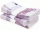The three workers received € 2,850 together for the work done. They divided them according to the time worked so that the first received 20% less than the second and the third € 50 more than the second. How much EUR did each worker receive?
• Two grandmothersTwo grandmothers went to sell eggs at the market, and they had a total of 100. When they sold all the eggs, they made the same money. The first grandmother says to the second: "If I sold my eggs for your price, I would earn 15 crowns. " The other grandmot
• Candy and boxesWe have some candy and empty boxes. When we put candies in boxes of ten, there will be 2 candies and 8 empty boxes left. When of eight, there will be 6 candies and 3 boxes left. How many candy and empty boxes left when we put candies in boxes of nine?
• Children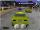Less than 20 children is played various games on the yard. They can create a pairs, triso and quartets. How many children were in the yard when Annie came to them?
• Two-digit numberIn a two-digit number, the number of tens is three greater than the number of units. If we multiply the original number by a number written in the same digits but in reverse order, we get product 3 478. Find the original number.
• Four pupilsFour pupils divided \$ 1485 so that the second received 50% less than the first, the third 1/2 less than a fourth, and fourth \$ 154 less than the first. How much money had each of them?
• The fourThe four pirates divided 65 coins to each other. They were sorted by age, the youngest receiving the least number of coins, each half more than the previous one. How many coins did the oldest pirate receive?
• Three workersThe company rewarded three workers CZK 9200, and the money was divided by the work they have done. The first worker to get twice than the second, the second three times more than the third. How much money did each worker receive?
• Box of chocolatesIn a box of chocolates were 16 candies. Christopher and Luke were distributed: a) Christopher had about 4 candies over Luke, b) Christopher had about 6 candies less than Luke, c) Christopher had 3 times more sweet than Luke. How many had each boy candies?
• PhotosThree friends Mirka, Lucka, and Hanka, collect photos. If Mirka had 3 more photos than she has, she would have as many as Hanka. If Lucka had 5 photos less than she has, she would be like Hanka. How many photographs does each have if they have 431 togethe
• Siblings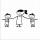Three siblings had saved up a total of 1,274 CZK. Peter had saved up to 15% more than Jirka and Hanka 10% less than Peter. How much money did they save each one of them?
• Tourists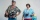Tourists hire a guide. Everyone will pay the same amount. If there are two less of them, they will pay 14 crowns more, and if there are three more, they will pay 14 crowns less. How many tourists are there and how much have the guides paid.
• MO Z8-I-1 2018Fero and David meet daily in the elevator. One morning they found that if they multiply their current age, they get 238. If they did the same after four years, this product would be 378. Determine the sum of the current ages of Fero and David.
• Friends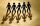Some friends had to collect the sum 72 EUR equally. If the three refused their part, others would have to give each 4 euros more. How many are friends?
• Stones 3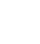Simiyu and Nasike each collected a number of stones in an arithmetic lesson. If Simiyu gave Nasike 5 stones, Nasike would have twice as many stones as Simiyu. If initially, Simiyu had five stones less than Nasike how many stones did each have?